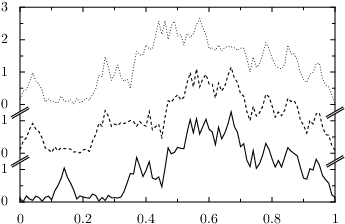# PyX — Gallery: graph/shift.py

0.7 KB
53.1 KB
3.6 KB
44.5 KB
20.6 KB
27.5 KB

## shift```# You can manually set the subaxes and exceed the axes ranges.
# The width of the regular axes can be modified by a size paramater.
# While most axes do not have a size parameter, it can be added to
# any existing axis very easily. For linear axes so called sizedlinear
# and autosizedlinear axes are defined by PyX already.

from pyx import *

subaxes = [graph.axis.linear(max=1),
graph.axis.linear(max=1),
graph.axis.sizedlinear(size=3, min=0, max=3)]

g = graph.graphxy(width=8, y=graph.axis.split(subaxes=subaxes))
g.plot([graph.data.file("shift.dat", x=1, y="i, \$(i+2)", context={"i": i})
for i in range(3)], [graph.style.line()])
g.writeEPSfile()
g.writePDFfile()
g.writeSVGfile()
```